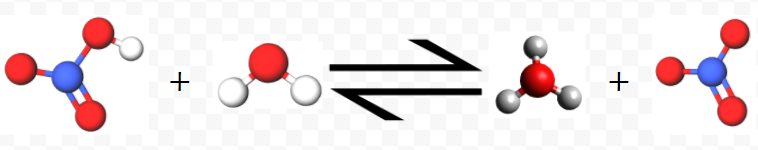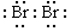# 11.6: Acids

A typical example of an acid is hydrogen chloride gas, HCl(g). When it dissolves in water, HCl reacts to form hydronium ions and chloride ions:

$\text{HCl}(g) + \text{H}_{2}\text{O}(l) \rightarrow \text{H}_{3}\text{O}^{+}(aq) + \text{Cl}^{-}(aq)$Thus the concentration of hydronium ions is increased above the value of 1.00 × 10–7 mol/L characteristic of pure water. Other acids, such as nitric acid, HNO3 behave in the same way:

$\text{HNO}_{3}(l) + \text{H}_{2}\text{O}(l) \rightarrow \text{H}_{3}\text{O}^{+}(aq) + \text{NO}_{3}^{-}(aq)$Thus the characteristic properties of solutions of acids are due to the presence of hydronium ions (or hydrogen ions). Whenever the concentration of hydronium ions exceeds 1.00 × 10–7 mol/L, an aqueous solution is said to be acidic. In 1884 a Swedish chemist, Svante Arrhenius (1859 to 1927), first recognized the importance of hydrogen ions. He defined an acid as any substance which increases the concentration of hydrogen (or hydronium) ions in aqueous solution.

The formation of a hydronium ion involves transfer of a proton from an acid molecule to a water molecule (as seen in the submicroscopic images above). This process is immediate―there are no free protons in solution which have left an acid molecule but have not yet attached themselves to a water molecule. To put it another way, a proton transfer is like a quarterback hand-off as opposed to a forward pass in foot- ball. The proton is always under the control and influence of one molecule or another. In the case of HCl we can indicate the transfer asAs the molecule collides with an H2O molecule, a hydrogen bond forms between the H and O atoms: Cl—H---OH2. When it begins to bounce away from the H2O molecule, the Cl atom loses control of the proton, leaving it attached to the O atom. The Cl atom retains control over both electrons which were in the H—Cl bond and thus ends up as a Cl ion. The H2O molecule ends up with an extra proton, becoming H3O+.

Example $$\PageIndex{1}$$ : Proton Transfer

Write balanced equations to describe the proton transfer which occurs when each of the following acids is dissolved in H2O:

1. HClO4 (perchloric acid) and
2. HBr (hydrogen bromide, or hydrobromic acid).

Solution:

Although a free proton is never actually produced in solution, it is often convenient to break the proton-transfer process into two hypothetical steps: (1) the loss of a proton by the acid, and (2) the gain of a proton by H2O.

a) When HClO4 loses a proton, H+, the valence electron originally associated with the H atom is left behind, producing a negative ion, ClO4. The proton can then be added to a water molecule in the second hypothetical step. Summing the two steps gives the overall proton transfer:

$$\text{HClO}_{4} \rightarrow \cancel{\text{H}^{+}} + \text{ClO}_{4}^{-}$$ step 1

$$\cancel{\text{H}^{+}} +\text{H}_{2}\text{O} \rightarrow \text{H}_{3}\text{O}^{+}$$ step 2
__________________________

$$\text{HClO}_{4} +\text{H}_{2}\text{O} \rightarrow \text{H}_{3}\text{O}^{+} + \text{ClO}_{4}^{-}$$ overall

b) Proceeding as in part a, we have

$$\text{HBr} \rightarrow \cancel{\text{H}^{+}} + \text{Br}^{-}$$ step 1

$$\cancel{\text{H}^{+}} +\text{H}_{2}\text{O} \rightarrow \text{H}_{3}\text{O}^{+}$$ step 2
______________________

$$\text{HBr} +\text{H}_{2}\text{O} \rightarrow \text{H}_{3}\text{O}^{+} + \text{Br}^{-}$$ overall

With practice, you should be able to write overall proton transfers without having to write steps 1 and 2 every time.

Another point to note about proton transfers is that in any equation involving ions, the sum of the ionic charges on the left side must equal the sum of the ionic charges on the right. For example, the last overall equation in Example 1 has HBr and H2O on the left. Neither is an ion, and so the sum of the ionic charges is zero. On the right we have H3O+ and Br, which satisfy the rule because +1 + (–1) = 0. An equation which does not satisfy this rule of charge balance will involve creation or destruction of one or more electrons and therefore cannot be valid. For example, the equation

$\text{2HBr} \rightarrow \text{2H}^{+} +\text{Br}_{2}$

cannot describe a valid proton transfer because the charges sum to zero on the left but +2 (because 2H+ ions) on the right. Careful examination reveals that there are 16 valence electrons (two octets in 2HBr) on the left but only 14 valence electrons (none in 2H+ and 14 in) on the right. Two electrons have been destroyed—something which cannot happen. Therefore the equation must be incorrect.

Because hydronium ions can be formed by transferring protons to water molecules, it is convenient when dealing with aqueous solutions to define an acid as a proton donor. This definition was first proposed in 1923 by the Danish chemist Johannes Brönsted (1879 to 1947) and the English chemist Thomas Martin Lowry (1874 to 1936). It is called the Brönsted-Lowry definition of an acid, and we will use it for the majority of this site. The Brönsted-Lowry definition has certain advantages over Arrhenius’ idea of an acid as a producer of H3O+(aq). This is especially true when acid strengths are compared, a subject we shall come to a bit later. Consequently, when we speak of an acid, we will mean a proton donor, unless some qualification, such as Arrhenius acid, is used.